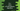# C++ program to find all prime numbers to n using sieve of Eratosthenes algorithm## C++ program to find all prime numbers to n using sieve of Eratosthenes algorithm:

In this post, we will learn how sieve of Eratosthenes algorithm works to find prime numbers up to n. The sieve of Eratosthenes is a popular and ancient algorithm to find all prime numbers to a given limit. It finds all prime numbers by marking all non-prime numbers.

We will learn the definition of sieve of Eratosthenes, how to use this algorithm and how to write a C++ program that uses sieve of Eratosthenes to find all prime numbers up to n. The program will take the value of n as an input from the user and print all prime numbers up to n.

### Sieve of Eratosthenes algorithm:

Sieve of Eratosthenes finds all prime numbers by iteratively marking non-prime values. It uses the below algorithm to find all prime numbers:

• Create a list of numbers from 2 to n.
• Start from the smallest prime number i.e. 2. Iterate through the list of numbers and mark all multiples of 2 as non-prime, i.e. it will mark 2, 4, 6… etc. as non prime in the list.
• Find the smallest number greater than 2 and not marked.

• If no number is found, stop.
• Else, mark all multiples of that number as non-prime up to n.
• When it ends, i.e. all non-prime numbers are marked, print out the prime numbers.

We can also start the scan from the square of a number and stop it if the square value is greater than n. Because, all the smaller multiples of that number are already marked as non-prime already. This will make the algorithm faster.

### Algorithm to use in the program:

We will use the below algorithm in the C++ program:

• Get the value of n as an input from the user.
• Declare an array of size n and initialize all elements of the array as 1.
• Run one loop with i from 2 to n - 1.

• Run one inner loop for j from i*i to n - 1. Increment the value by i after each iteration.
• Assign the j - 1 value as 0.
• Once the loop is completed, iterate through the array from i = 1 to length - 1. If the value in the array is 1, print that value, i.e. it is a prime number.

### C++ program:

Below is the complete C++ program:

``````#include <iostream>
#include <vector>

using namespace std;

void printPrime(int n)
{
vector<int> nums(n, 1);

for (int i = 2; i < n; i++)
{
for (int j = i * i; j < n; j += i)
{
nums[j - 1] = 0;
}
}

for (int i = 1; i < n; i++)
{
if (nums[i - 1] == 1)
{
cout << i << " ";
}
}
}

int main()
{
int n;
cout << "Enter the value of n" << endl;

cin >> n;

printPrime(n);

return 0;
}``````

Here,

• We used a vector instead of an array.
• The value of n is stored in the integer variable n.
• printPrime method is used to print all the prime numbers from 1 to n.
• We are using a vector of size n and initializing the values of it as 1.
• The first two for loops are used to mark the non-prime values as 0.
• The last for loop is used to print all prime values. It checks the array content and if it is 1, it prints the value of i.

If you run this program, it will print output as like below:

``````Enter the value of n
40
1 2 3 5 7 11 13 17 19 23 29 31 37``````